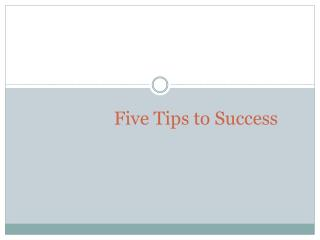DownloadDownload PresentationFive Tips to Success

# Five Tips to Success

Télécharger la présentation## Five Tips to Success

- - - - - - - - - - - - - - - - - - - - - - - - - - - E N D - - - - - - - - - - - - - - - - - - - - - - - - - - -
##### Presentation Transcript

1. Five Tips to Success

2. Work hard

3. Try more exercises and more practice

4. Do the labs and assignments yourself

5. Be patient with the machine

6. If you really need that, do it quietly ... But not in Class. What you have to do is just inform me about your situation and I will not mark your absent.

7. What is C? • C is a programming language. • It was developed in 1972 USA. • It was designed and written by a man named dennis ritchie. • C is the base for all computer languages like C++, java etc. • Major parts of popular operating systems like Windows, UNIX, Linux are still written in C.

8. Getting Started with C • Communicating with a computer involves speaking the language the computer under stands. • As in learning of English language first we learn English alphabets, which forms a word, words combine to form a sentence and sentences forms a paragraph. • Like English language we must have to learn alphabets, numbers and symbols which are used in C.

9. Steps in learning English language • Alphabets • Words • Sentences • Paragraphs

10. Steps in learning C • Alphabets, Digits, Special symbol • Constants, Variables, Keywords • Instructions • Programs

11. The C character Set • A character denotes any alphabet, digit or special symbol used to represent information.

12. Constants, Variables and Keywords • The alphabets, numbers and special symbols when properly combine form constants ,variables and keywords. • Any entity that doesn`t change is called constant. • Any entity that may change is called variable.

13. X X 3 is stored in a memory location and a name X is given to it. we assign a new value 5 to the same memory location X. So the location X can Hold different values. 3 and 5 cannot change.

14. Types of C constants C constants can be divided into two major categories: • Primary constants • Secondary Constants These are further categorized as

15. Rules for constructing Integer constants • An integer constant must have at least one digit. (b) It must not have a decimal point. (c) It can be either positive or negative. (d) If no sign precedes an integer constant, it is assumed to be positive. (e) No commas or blanks are allowed within an integer constant.

16. (f) The allowable range for integer constants is -32768 to 32767. • The range of integer constant depend upon the compiler. for a 16-bit complier like Turbo C or Turbo C++ the range is -32768 to 32767. working with a 16-bit complier • Ex: 426 +782 -8000 -7605

17. Rules for Constructing Real Constants • Real constants are often called Floating Point constants. • It may be in fractional and Exponential form. • Following rules must be followed for constructing real constants expressed in fractional form:

18. A real constant must have at least one digit. (b) It must have a decimal point. (c) It could be either positive or negative. (d) Default sign is positive. (e) No commas or blanks are allowed within a real constants. Ex:+325.34 426.0 -32.76 -48.5792

19. The exponential form of representation of real constants is usually used if the value of the constant is either too small or too large. • The real constant is represented in two parts . The part appearing before “e” is called mantissa and the part following “e” is called exponent. • 0.000342 can be represented in exponential form as 3.42e-4. • Following rules must be observed by constructing constants expressed in exponential form:

20. The mantissa part and the exponential part should be separated by a letter e or E. (b) The mantissa part may have a positive or negative sign. (c) Default sign of mantissa part is positive. (d) The exponent must have at least one digit, must be a positive or negative integer. Default sign is positive. (e) Range of real constants expressed in exponential form is -3.4e38 to 3.4e38. Ex: +3.2e-5 4.1e8 -0.2E+3 -3.2e-5

21. Rules for constructing Character Constants • A character constant is a single alphabet, single digit or a single special symbol enclosed within single inverted commas. Both the inverted commas should point to the left. For example `a` is a valid character constant whereas `A` is not. (b) The maximum length of a character constant can be 1 character. Ex: `A` `I` `5` `=`

22. The End